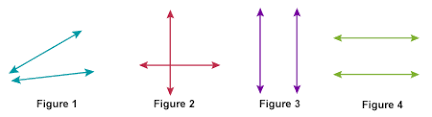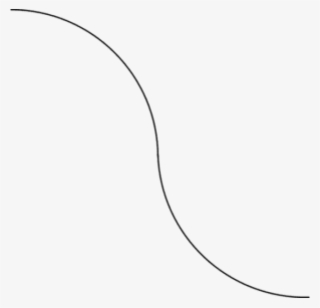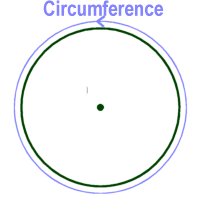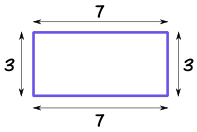# Lines in Mathematics

### Lines in Mathematics:

Lines are quite important in mathematics because having a good understanding of them would help students to better understanding angles. Every form of line must begin from a point. In fact, a line can only be drawn within two different points.

Just think of a point as a location.

A point has no length, depth or width. It is generally represented as a dot.

Note: A point is not necessarily a dot. A dot shows you where the point (location) really is.

Below are 5 different points; represented by a dot.

. . . . .

A line can be defined as the path between two points. A line usually have a length but may have no width.

Lines can be generally put into 2 categories in mathematics. Every other type of line will lie within these 2. The 2 categories of lines are Straight Lines and Curved Lines.

• ### Straight line

When the path between two points are straight, then the line that is associated with the path is a straight line. A straight line can be drawn in any direction. It could go from north to south or east to west. The image below shows a straight line running from east to west.

EAST ______________________ WEST

Below are types of straight line

#### 1. Horizontal Line

A horizontal line is a line that runs from left to right or east to west in a straight line.

#### 2. Vertical Line

A vertical line runs from top to bottom or north to south in a straight line.

#### 3. Perpendicular line

The word perpendicular simply means “at right angles”.

Two lines are said to be perpendicular if they meet or cross each other at a right angle.

Note: A right angle is an angle at ninety degrees (90o).Image Credit: Wyzant

In the diagram above, you will observe that the line CD meets line AB at point E, which is at a right angle (90o). Line CD is therefore said to be perpendicular to line AB at point E.

Note the followings in the above diagram:

• Line AB is a HORIZONTAL LINE.
• Line CD is a VERTICAL LINE.
• If Line CD had met line AB at another angle; lets say at angle 45o, then we can correctly say that line CD meets line AB OBLIQUELY.

#### 4. Parallel Line

Two lines are considered to be parallel if they will never meet each other, no matter the length at which you extend them. The diagram below show two parallel lines.Image Credit: Tes

#### 5. Oblique Line

Oblique lines are neither perpendicular nor parallel. They are slanted or tilted lines that will finally meet at some point, but not at a right angle (90o).In the diagram above, the lines in:

• Figure 1 is oblique
• Figure 2 is perpendicular
• Figures 3 and 4 are parallel

Note: Oblique lines are sometimes referred to as slanting lines.

• ### Curved line

When the path between two lines isn’t straight, then such line can be said to be curved. Below is a curved line.Image Credit: PngkeyAlfred Ajibola is a Medical Biochemist, a passionate Academician with over 7 years of experience, a Versatile Writer, a Web Developer, a Cisco Certified Network Associate and a Cisco CyberOps Associate.

#### NOTABLE POINTS IN Mathematics Circumference in mathematics means the distance around the edge of a circle (or a another curverd surface). Perimeter means the distance around a square or rectangle or polygon. Circumference is to circle or any other curved surfaceImage Credit: Ducksters Perimeter is to square or rectangle or polygonImage Credit: Maths is Fun

CONTRIBUTE TO THIS TOPIC | ASK A QUESTION